## Sunday, January 10, 2021

### Electricity at Power Plants: UNIZOR.COM - Physics4Teens - Electromagneti...

Notes to a video lecture on http://www.unizor.com

Electricity at Power Plants

Addressing distribution of electricity, we will primarily discuss the way electricity, produced at the power plants, is delivered to consumers.
We will concentrate on the process of distribution of electricity generated from the kinetic energy of rotating turbines, as the most quantitatively significant source of electricity.

Three stages of this distribution are
(a) at the power plant
(b) in transit
(c) at consumers.

This lecture is about what's going on at the power plant that produces the electricity from the kinetic energy of rotating turbines.

Turbines at electric power plants are rotated because of a flow of steam or water, or wind. Turbines are acting as rotors in the electric power generator, while the electricity is produced in stators based on the principles of electromagnetic induction.

Hydroelectric Stations

Let's estimate theoretically an amount of energy that a hydroelectric station can produce.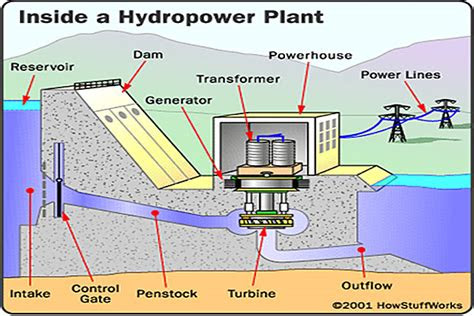First important component in generating electricity at a hydroelectric station is falling water. We can have falling water by building a dam on a river, like Hoover Dam on Colorado river or use natural difference in the level of water of the waterfalls, like at Niagara falls.

Having the water at two levels, we should direct the flow from top to bottom onto turbines through pipes. Amount of electricity that can be generated obviously depends on the amount of potential energy water at the top has relatively to the bottom level. As the water falls through the pipes onto turbine, its potential energy is converted into kinetic energy of moving water. This is how much energy we can use to generate electricity. It depends on the amount of water flowing through pipes and the height difference between the top and the bottom levels.

Let's assume that the amount of water falling down through pipes from top to bottom level is M (kg/sec) and the difference in height from top to bottom is H (m).
That means that the amount of energy falling water is losing per unit of time is
Pwater = M·g·H (J/sec or W)

Then we have to solve a purely technical problem to convert this energy into rotational energy of turbines.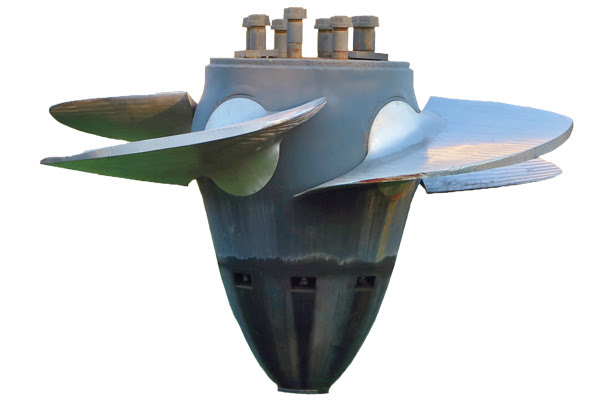Different designs of turbines have been used and tested during a long time of using hydroelectric power. Contemporary turbines are pretty efficient in this process of conversion, but still far less than 100% effective. Losses of energy always exist, and we need some coefficient of efficiency of a turbine to get exact amount of rotational energy produced by falling water.
Let's assume that k is such a coefficient. It has a value from 0 (absolutely ineffective conversion) to 1 (full energy amount of falling water is converted into rotational energy of a turbine). Then the amount of rotational energy produced by turbines per unit of time is
Pturbine = k·M·g·H

Next step is to convert rotational energy of turbines into electric energy.
This is done by generators, which we discussed in previous lectures.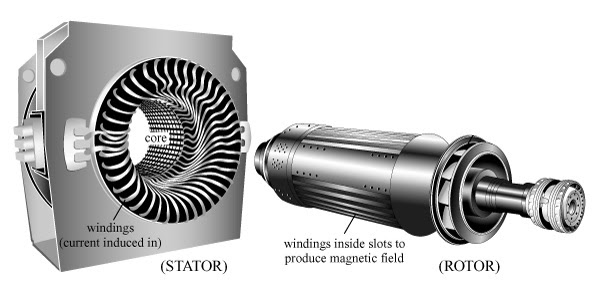The contemporary generators are pretty effective with norm being above 90%, so we can assume that the coefficient of effectiveness k introduced above encompasses both effectiveness of converting energy of falling water into rotation of turbines and conversion of rotation into electric energy.
So, overall energy produced by a hydroelectric power station per unit of time (that is, the power produced) is
P = k·M·g·H
The hydroelectric power stations can be very large and can produce a lot of electric energy. The most powerful electric power stations are hydroelectric. The problem is, there are not too many rivers suitable for building hydroelectric power stations and an environmental effect of building a hydroelectric power station can be significant.

At the same time, the hydroelectric power stations are pretty efficient, the coefficient k in the formula above can be above 0.8, which means that about 80% of the power of water falling on turbines is effectively converted into electric power.

Coal Burning Stations

Almost a third of electricity generated in the world is produced by fossil fuel burning power stations.
Let's examine the coal burning power station.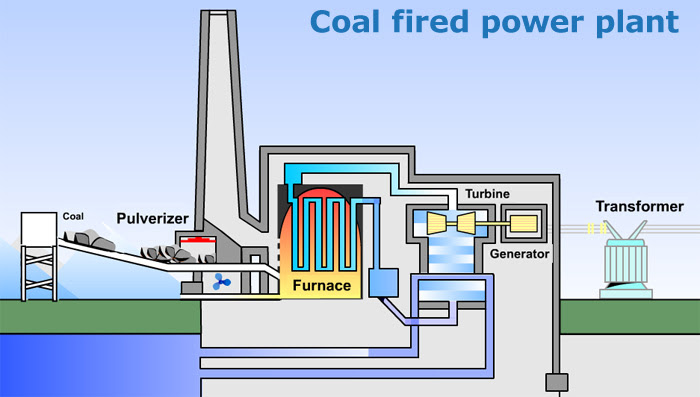The main steps of producing electricity by burning fossil fuel are
(a) burning fossil fuel to boil water, converting chemical energy of burning fuel into kinetic energy of produced steam,
(b) converting kinetic energy of steam into rotation of turbines,
(c) converting rotational energy of turbines into electricity by generators.

Coal is a major source of fossil fuel with natural gas and oil following.
Convenience of putting a coal burning electric power station anywhere should be weighed against environmental impact of such a plant.

Producing energy from burning coal is not a very efficient way to extract chemical energy. Significant portion of the energy produced by burning coal is wasted on each step and the overall efficiency of such a power station is about 40%. Most of the energy losses occur during the first stage of generating electricity - burning coal to boil water and produce steam.
Some efficiency can be achieved by pulverizing coal to powder. However, the main product of burning fossil fuel - carbon dioxide CO2 - produces some unavoidable negative environmental effect.

Nuclear Power Plants

The difference between a nuclear power plant and coal burning one is at the first stage to boil the water. While at coal burning plants the source of heat to boil water is burning coal, at the nuclear power plant the source of heat is energy released by breaking nuclei of heavy elements, like Uranium or Plutonium, into lighter components using neutrons.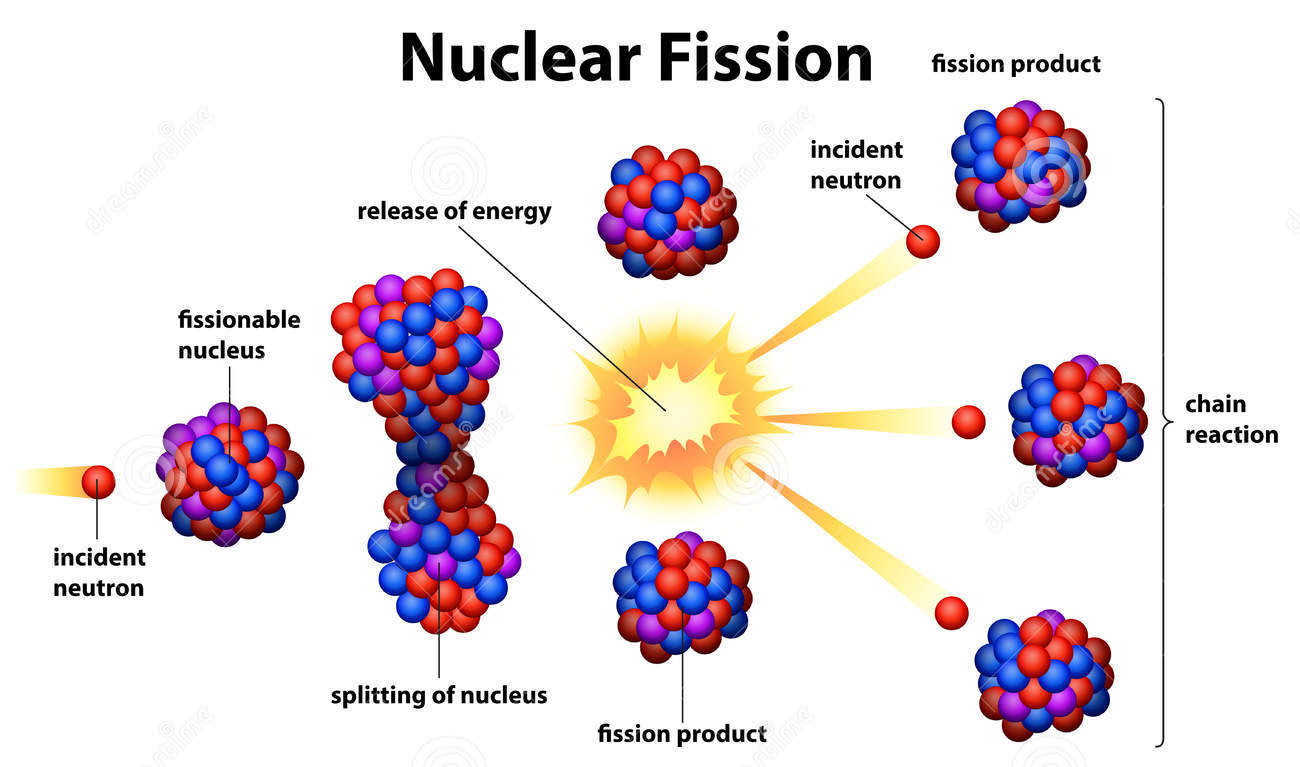The main steps of producing electricity in a nuclear power plant are
(a) bombarding the enriched radioactive material (Uranium, Plutonium or other) with neutrons causing the nuclei of this material to break, releasing certain amount of heat to boil water getting steam,
(b) converting kinetic energy of steam into rotation of turbines,
(c) converting rotational energy of turbines into electricity by generators.

Efficiency of nuclear power plants is quite limited inasmuch as in coal burning power stations and is about 40%. That is, about 40% of the energy generated by heat is converted into electricity.

Of interest is a process of nuclear fission that produces the heat. Here is a simplified model of this process.

When a nucleus of Uranium-235 (92 protons + 143 neutrons) is bombarded with a neutron, it temporarily accepts this neutron inside, becoming Uranium-236 (92 protons + 144 neutrons).
This isotope is not stable and a nucleus breaks into different parts. This is a complex process and parts might be different.

A typical scenario might be as follows.
Broken parts are Barium (56 protons + 83 neutrons), Krypton (36 protons + 58 neutrons) and 3 neutrons are released to bombard other nuclei of Uranium 235, causing a chain reaction.

The combined mass of all parts is less than the mass of initial components. The remaining mass of an unstable nucleus of Uranium-236 is converted into radiation (heat, gamma-rays). The heat is used to boil the water, converting it into high energy steam to rotate the turbines.

The corresponding equations describing nuclear fission is:
1n0 + 235U92236U92
(fission)
139Ba56 + 94Kr36 + 31n0 + γ

In reality the process is much more complex because the broken parts of a nucleus might be different, themselves not stable and further emitting elementary particles.
The process must be controlled by reducing the number of neutrons flying in all the directions after fission to prevent a nuclear explosion.